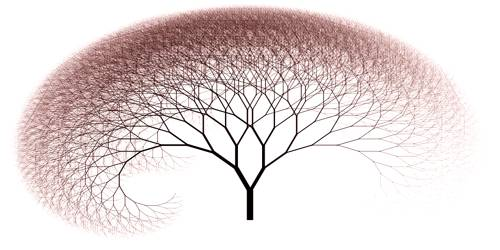Technology

# Recursive in Computer ScienceRecursive in Computer Science

Recursion is an important concept in computer science. When a function calls itself, it is called a recursive function. Using a recursive algorithm, certain problems can be solved quite easily. In the easy word, recursion is the process of breaking down a problem into smaller pieces that can be solved easily, then apply the same procedure to each piece and then combine the results. It is one of the fundamental tools of computer science. It can be helpful in other calculations, such as calculating Fibonacci numbers or the maximum common divisor.

Recursive functions are common in computer science because they allow programmers to write proficient programs using a minimal amount of code. Recursive programming is directly related to mathematical induction, a technique for proving facts about natural numbers. While the concept of recursive programming can be difficult to grasp initially, mastering it can be very useful.

A recursive procedure must have two properties as follows.

• There must be certain arguments, called “base criteria”, for which the procedure does not call itself.
• Each time the procedure does call itself; it must be closer to the base criteria.